Like   Tweet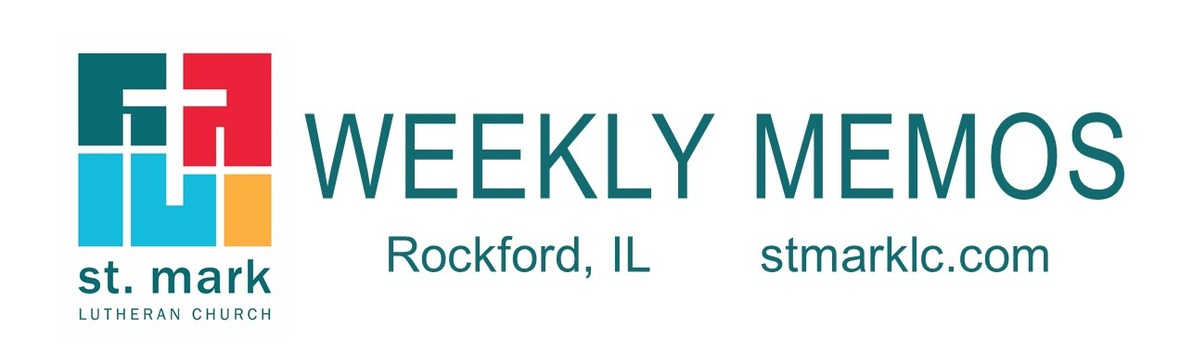table div table+table div table{width:100%;padding:0}table div table+table div table img{width:96.23%;padding:0;float:none}table div table+table div table td{width:100%;padding:0 1.88% 18px}/* styles */## This Week at St. Mark August 7 - 14, 2022

 table div table+table+table+table div table{width:100%;padding:0}table div table+table+table+table div table img{width:96.23%;padding:0;float:none}table div table+table+table+table div table td{width:100%;padding:0 1.88% 18px}/* styles */## Sunday, August 7 Pastor Woody Knutson Preaching

9:00 A.M. Worship with Live-Stream
10:00 A.M. Fellowship Hour with coffee and donuts
11:00 A.M. Outdoor Worship on East Lawn

## Tuesday, August 9

10:30 A.M. St. Mark Duplicate Bridge
5:30 P.M. Children's Ministry

## Thursday, August 11

9:00 A.M. Cherry Valley Bridge Group
12:30 P.M. Sew and Sews
6:30 P.M. Emotions Anonymous

## Friday, August 12

7:30 A.M. St. Mark Breakfast Group

## Sunday, August 14 Back to School Blessings

9:00 A.M. Worship with Live-Stream
10:00 A.M. Fellowship Hour with coffee and donuts
11:00 A.M. Outdoor Worship on East Lawn

 table div table+table+table+table+table+table div table{width:100%;padding:0}table div table+table+table+table+table+table div table img{width:96.23%;padding:0;float:none}table div table+table+table+table+table+table div table td{width:100%;padding:0 1.88% 18px}/* styles */## News and Events

 table div table+table+table+table+table+table+table+table div table{width:100%;padding:0}table div table+table+table+table+table+table+table+table div table img{width:96.23%;padding:0;float:none}table div table+table+table+table+table+table+table+table div table td{width:100%;padding:0 1.88% 18px}/* styles *//* styles */ August 7, 2022 - 9th Sunday after Pentecost 9:00 am - Traditional Worship in the Sanctuary (Prelude will begin at 8:55) 10:00 am - Fellowship hour with coffee and donuts 11:00 am - Praise Service in Fellowship Hall Our weekly live-stream will occur during the 9:00 am Sanctuary service. Follow this link for the live-stream service Sunday.
 table div table+table+table+table+table+table+table+table+table+table div table{width:100%;padding:0}table div table+table+table+table+table+table+table+table+table+table div table img{width:96.23%;padding:0;float:none}table div table+table+table+table+table+table+table+table+table+table div table td{width:100%;padding:0 1.88% 18px}/* styles *//* styles */ You're Invited! Pastor Katrina, Melinda Alekna and Becca Honzel went to area fire departments and a police station to invite them to our Septemberfest weekend. They thanked them for all they do for our community every day! We will be honoring our first responders with a catered lunch on Sunday, September 11.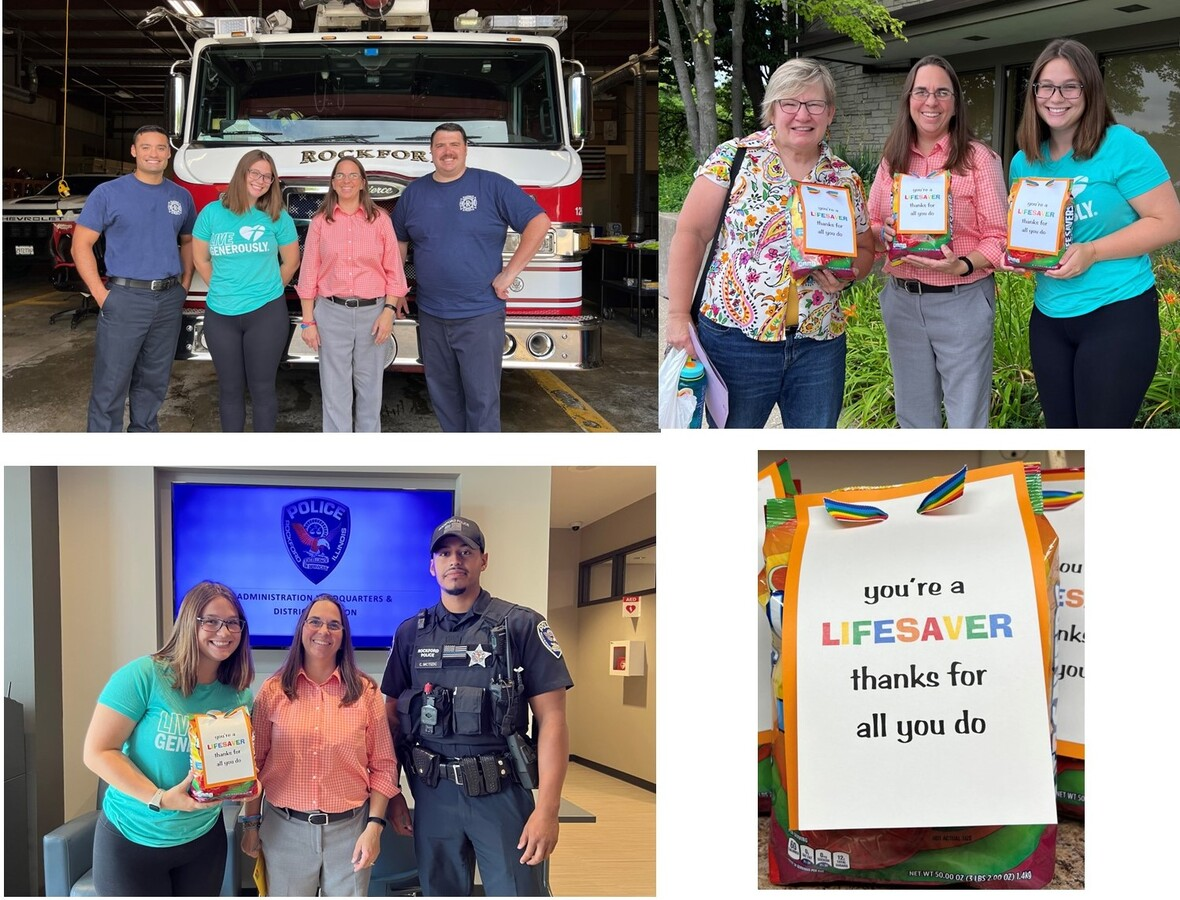table div table+table+table+table+table+table+table+table+table+table+table+table+table div table{width:100%;padding:0}table div table+table+table+table+table+table+table+table+table+table+table+table+table div table img{width:96.23%;padding:0;float:none}table div table+table+table+table+table+table+table+table+table+table+table+table+table div table td{width:100%;padding:0 1.88% 18px}/* styles */Septemberfest
Friday - Sunday, September 9-11! A weekend of celebration.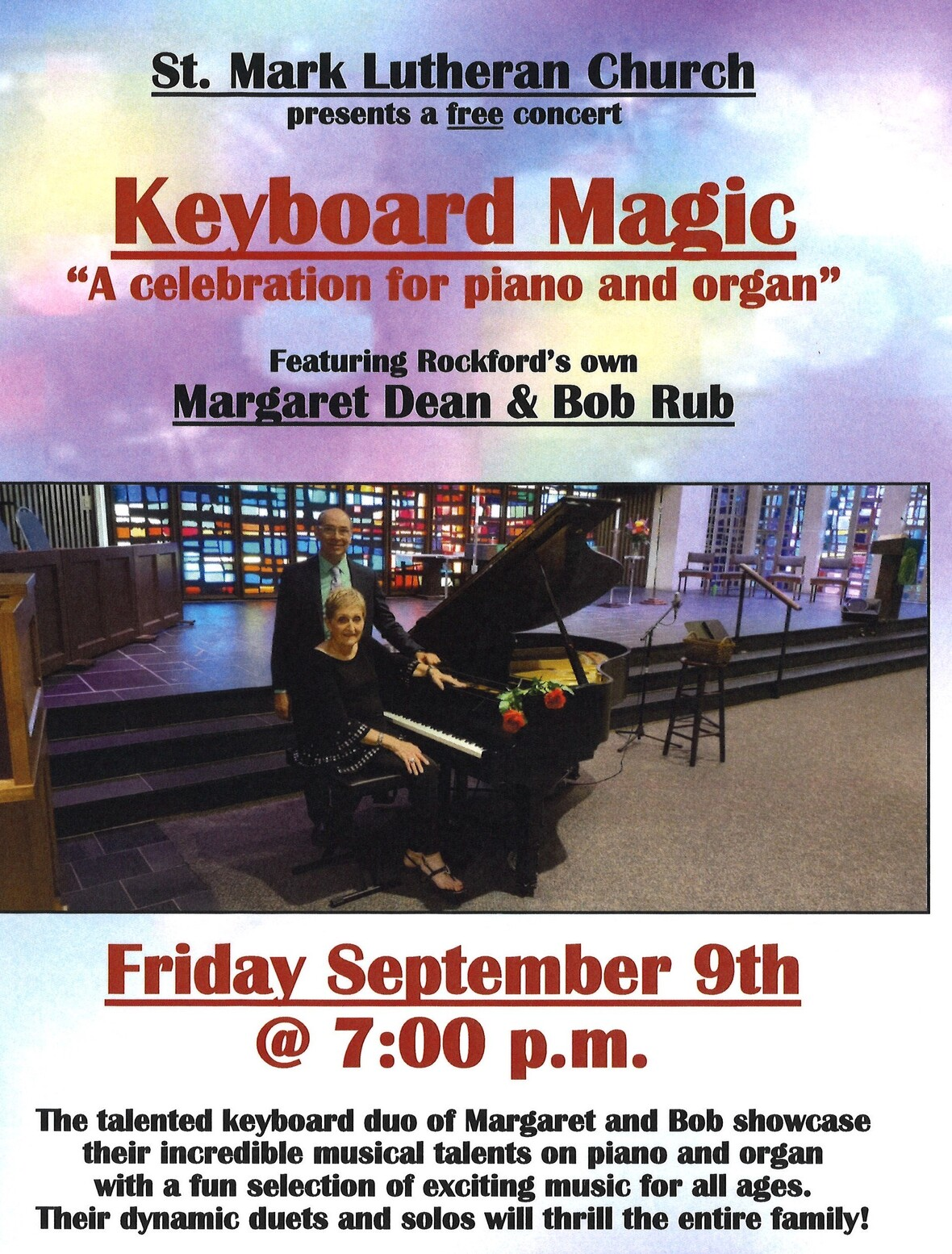table div table+table+table+table+table+table+table+table+table+table+table+table+table+table+table+table div table{width:100%;padding:0}table div table+table+table+table+table+table+table+table+table+table+table+table+table+table+table+table div table img{width:96.23%;padding:0;float:none}table div table+table+table+table+table+table+table+table+table+table+table+table+table+table+table+table div table td{width:100%;padding:0 1.88% 18px}/* styles */Lasagna Love - Meal Assembly Saturday, September 10th
Sign up for an hour shift or more for Saturday, September 10th. The hours are from 10:00 a.m. to 1:00 P.M. We will be making, assembling, and preparing lasagna! We need volunteers! Individuals, groups, families, co-workers, etc. We plan to make enough lasagna to feed Rock House Kids, Carpenter’s Place, Rescue Mission, and others. Fun, friendly, foodie, event. P.S. got an apron? Wear it! Sign-up starts now! Call the church office or sign-up on Sunday mornings.

 table div table+table+table+table+table+table+table+table+table+table+table+table+table+table+table+table+table+table div table{width:100%;padding:0}table div table+table+table+table+table+table+table+table+table+table+table+table+table+table+table+table+table+table div table img{width:96.23%;padding:0;float:none}table div table+table+table+table+table+table+table+table+table+table+table+table+table+table+table+table+table+table div table td{width:100%;padding:0 1.88% 18px}/* styles */Septemberfest - Sunday, September 11!
All are Welcome! We will have one worship service this morning at 10:30 a.m. outside under the tent. Worship, music, fellowship, food, and celebration!

There will be designated seating for those who have mobility issues and plenty of ushers to help. Following the service there will be a catered lunch, the petting zoo will be back, and some activities.

We encourage you to invite friends and family to our weekend of celebration and opportunity.

 table div table+table+table+table+table+table+table+table+table+table+table+table+table+table+table+table+table+table+table+table div table{width:100%;padding:0}table div table+table+table+table+table+table+table+table+table+table+table+table+table+table+table+table+table+table+table+table div table img{width:96.23%;padding:0;float:none}table div table+table+table+table+table+table+table+table+table+table+table+table+table+table+table+table+table+table+table+table div table td{width:100%;padding:0 1.88% 18px}/* styles */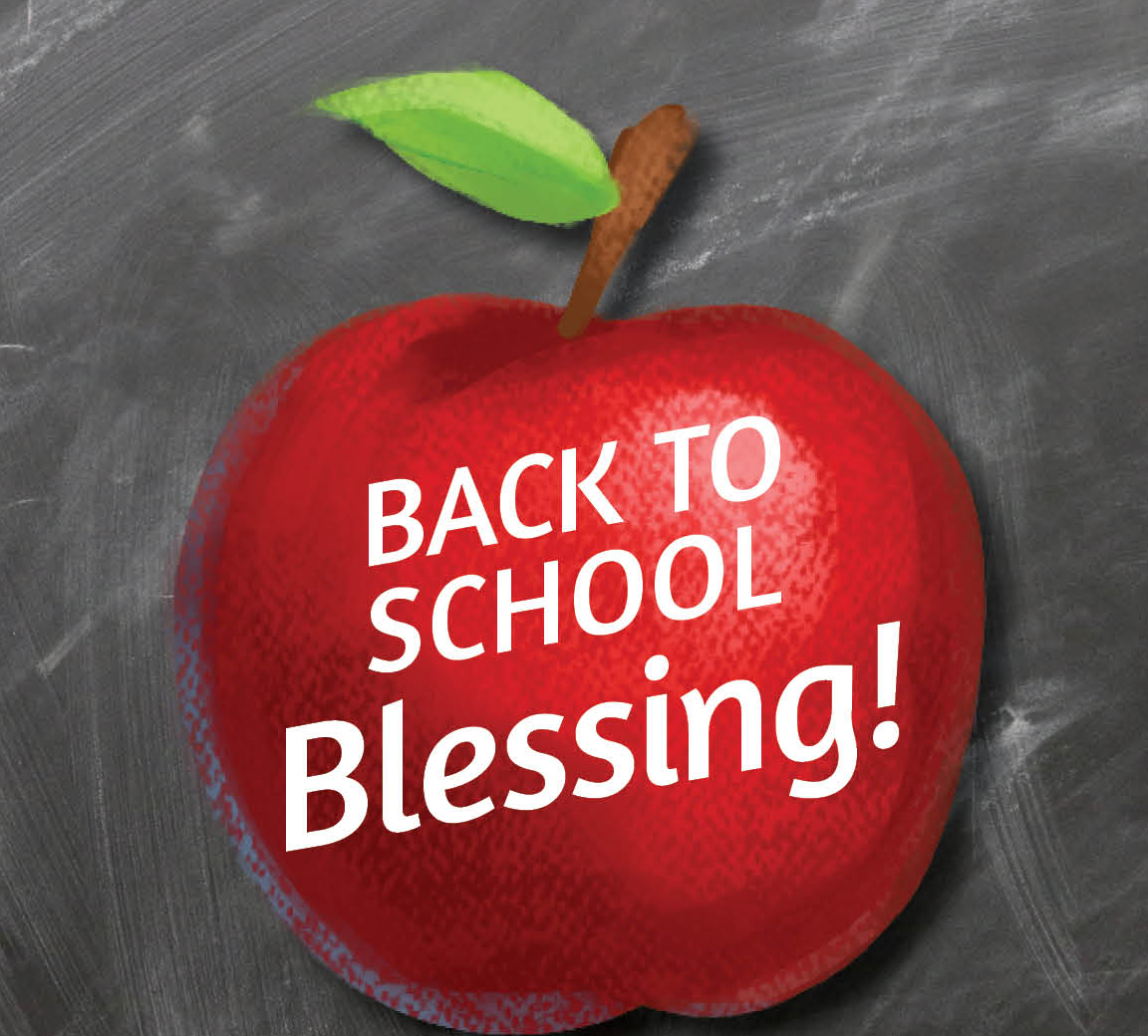Back to School Blessing
August 14 and 28, St. Mark will provide a back to school blessing for anyone returning to school during both services.

 table div table+table+table+table+table+table+table+table+table+table+table+table+table+table+table+table+table+table+table+table+table+table div table{width:100%;padding:0}table div table+table+table+table+table+table+table+table+table+table+table+table+table+table+table+table+table+table+table+table+table+table div table img{width:96.23%;padding:0;float:none}table div table+table+table+table+table+table+table+table+table+table+table+table+table+table+table+table+table+table+table+table+table+table div table td{width:100%;padding:0 1.88% 18px}/* styles */Women's Bible Study
The Women's Wednesday Morning Bible Study will resume on September 7th, 2022. We will meet at the Stockholm Inn for breakfast at 9:00 for good food and good fellowship. This is open to all women at St. Mark and their friends. This year we will begin with a 6-week study on Honoring Our Neighbor's Faith. We will discuss Buddhism, Hinduism, and Judaism. We will then begin a Bible Study on the book of Ecclesiastes from the Old Testament. If time permits we will finish the year studying the book of Romans. Our regularly scheduled Bible study will begin on September 28th. It is on this date that we will begin the study of Buddhism. The Bible Study is every Wednesday morning from 9:00-10:00 in the conference room. Please join us if you can as we study God's Word with discussion, sharing of life experiences, and prayer! If you have any questions call Deb Iverson (815-218-5152) or email at dsiverson5@gmail.com.

 table div table+table+table+table+table+table+table+table+table+table+table+table+table+table+table+table+table+table+table+table+table+table+table+table div table{width:100%;padding:0}table div table+table+table+table+table+table+table+table+table+table+table+table+table+table+table+table+table+table+table+table+table+table+table+table div table img{width:96.23%;padding:0;float:none}table div table+table+table+table+table+table+table+table+table+table+table+table+table+table+table+table+table+table+table+table+table+table+table+table div table td{width:100%;padding:0 1.88% 18px}/* styles */The Sew & Sew group is looking for new members.
We meet on the second and fourth Thursdays of each month from 12:30 to 3:00 PM. Don’t be scared away by the fact that we sew and make quilts. You don’t have to be able to sew because there are several steps in putting together quilts or port pillows that do not require sewing. Some of us take fabric home and work on quilt tops at home and then bring it back to church to finish the quilt with the batting and backing. The best part is our super machine quilter Chris Allen takes a pinned quilt home and brings it back all beautifully quilted. Then we have members that like to do hand stitching, so they sit and hand sew the binding to the back of the quilt along with our labels that read, "Made with love by St. Mark Lutheran Sew and Sews".

We make quilts for each member of the families that move into Habitat for Humanity homes, and quilts for foster children. We make soft fleece throws for the children at Rock House Kids, and port pillows for cancer patients to use on their car seat belts.

 table div table+table+table+table+table+table+table+table+table+table+table+table+table+table+table+table+table+table+table+table+table+table+table+table+table+table div table{width:100%;padding:0}table div table+table+table+table+table+table+table+table+table+table+table+table+table+table+table+table+table+table+table+table+table+table+table+table+table+table div table img{width:96.23%;padding:0;float:none}table div table+table+table+table+table+table+table+table+table+table+table+table+table+table+table+table+table+table+table+table+table+table+table+table+table+table div table td{width:100%;padding:0 1.88% 18px}/* styles */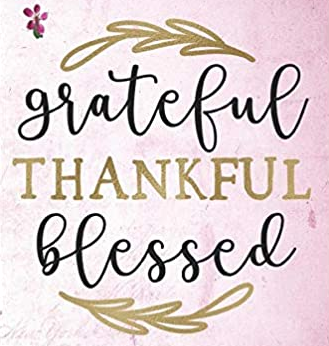Our deep appreciation and kind expressions of sympathy for all your cards, memorial gifts, and prayers in helping to make my wife's funeral service a beautiful farewell. Janet was a caring person and loved people. We had 63 years together, I loved her and I will miss her, but I know that Jesus lives, there is an Easter, and a place for Janet to rest in the resurrection. Bless all of you. Pastor Don Lavelle and family

 table div table+table+table+table+table+table+table+table+table+table+table+table+table+table+table+table+table+table+table+table+table+table+table+table+table+table+table+table div table{width:100%;padding:0}table div table+table+table+table+table+table+table+table+table+table+table+table+table+table+table+table+table+table+table+table+table+table+table+table+table+table+table+table div table img{width:96.23%;padding:0;float:none}table div table+table+table+table+table+table+table+table+table+table+table+table+table+table+table+table+table+table+table+table+table+table+table+table+table+table+table+table div table td{width:100%;padding:0 1.88% 18px}/* styles */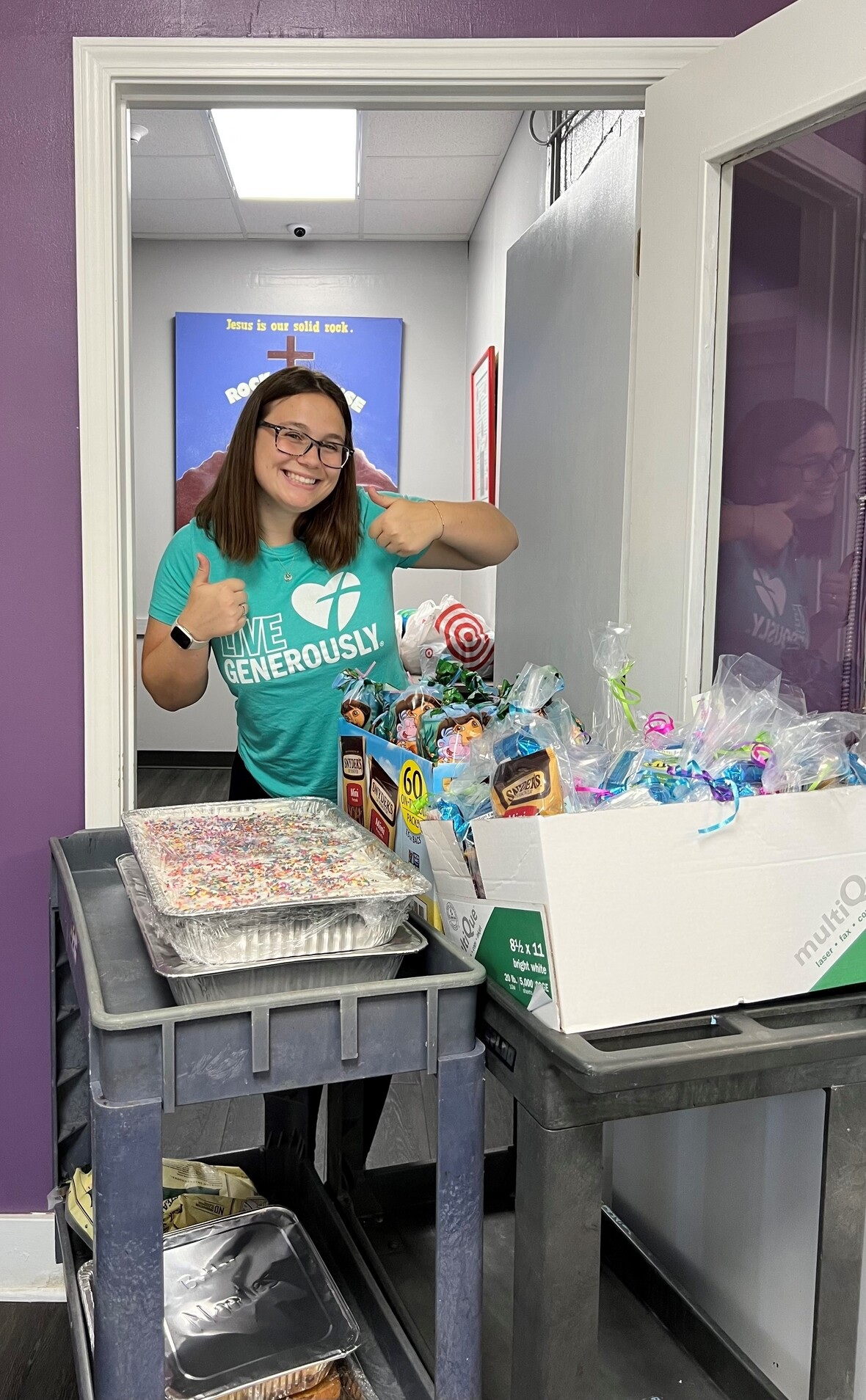/* styles */ Sign-up to make a meal for Rock House kids. We need September and October commitments. Ask your friends, Co-workers, and family to help. We have ideas and recipes! E-mail or call Melinda Alekna 815-871-0390,melinda@stmarklc.com.
 table div table+table+table+table+table+table+table+table+table+table+table+table+table+table+table+table+table+table+table+table+table+table+table+table+table+table+table+table+table+table+table div table{width:100%;padding:0}table div table+table+table+table+table+table+table+table+table+table+table+table+table+table+table+table+table+table+table+table+table+table+table+table+table+table+table+table+table+table+table div table img{width:96.23%;padding:0;float:none}table div table+table+table+table+table+table+table+table+table+table+table+table+table+table+table+table+table+table+table+table+table+table+table+table+table+table+table+table+table+table+table div table td{width:100%;padding:0 1.88% 18px}/* styles */Nursery News!
The nursery is during the 9:00 service! Children are and always will be welcome in worship here at St. Mark, but we also recognize that nursery care can be a helpful option.

For now, we will be relying on volunteers to serve as nursery attendants. You can sign up here: Nursery Volunteer Sign-Up. Adults and youth are welcome to volunteer, but youth under 16 need to serve in pairs. There may be times when the nursery is open but no volunteer is available. In this case, you are still welcome to use the space to play or relax with your child. We hope you find these options helpful! We are always looking for ways to better support our members and visitors. If you have questions or want more information, please email julie@stmarklc.com.

 table div table+table+table+table+table+table+table+table+table+table+table+table+table+table+table+table+table+table+table+table+table+table+table+table+table+table+table+table+table+table+table+table+table div table{width:100%;padding:0}table div table+table+table+table+table+table+table+table+table+table+table+table+table+table+table+table+table+table+table+table+table+table+table+table+table+table+table+table+table+table+table+table+table div table img{width:96.23%;padding:0;float:none}table div table+table+table+table+table+table+table+table+table+table+table+table+table+table+table+table+table+table+table+table+table+table+table+table+table+table+table+table+table+table+table+table+table div table td{width:100%;padding:0 1.88% 18px}/* styles */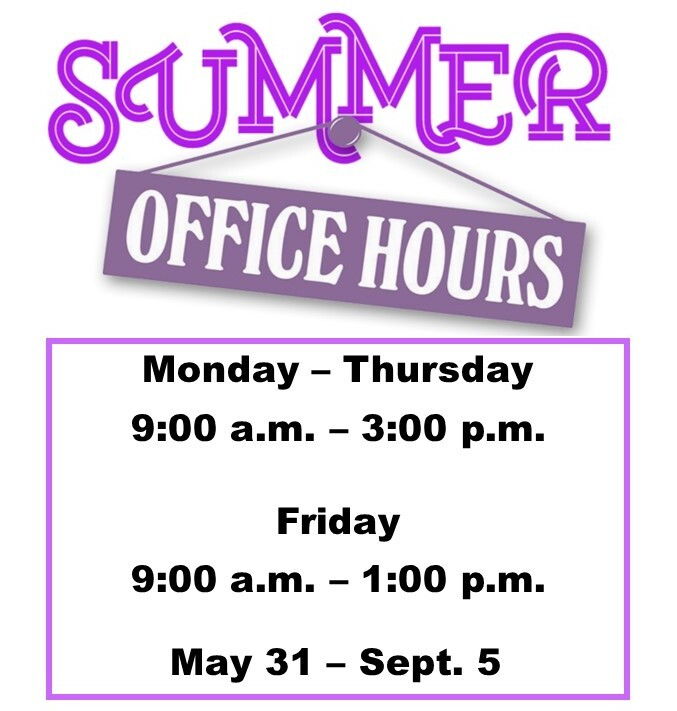table div table+table+table+table+table+table+table+table+table+table+table+table+table+table+table+table+table+table+table+table+table+table+table+table+table+table+table+table+table+table+table+table+table+table+table div table{width:100%;padding:0}table div table+table+table+table+table+table+table+table+table+table+table+table+table+table+table+table+table+table+table+table+table+table+table+table+table+table+table+table+table+table+table+table+table+table+table div table img{width:96.23%;padding:0;float:none}table div table+table+table+table+table+table+table+table+table+table+table+table+table+table+table+table+table+table+table+table+table+table+table+table+table+table+table+table+table+table+table+table+table+table+table div table td{width:100%;padding:0 1.88% 18px}/* styles */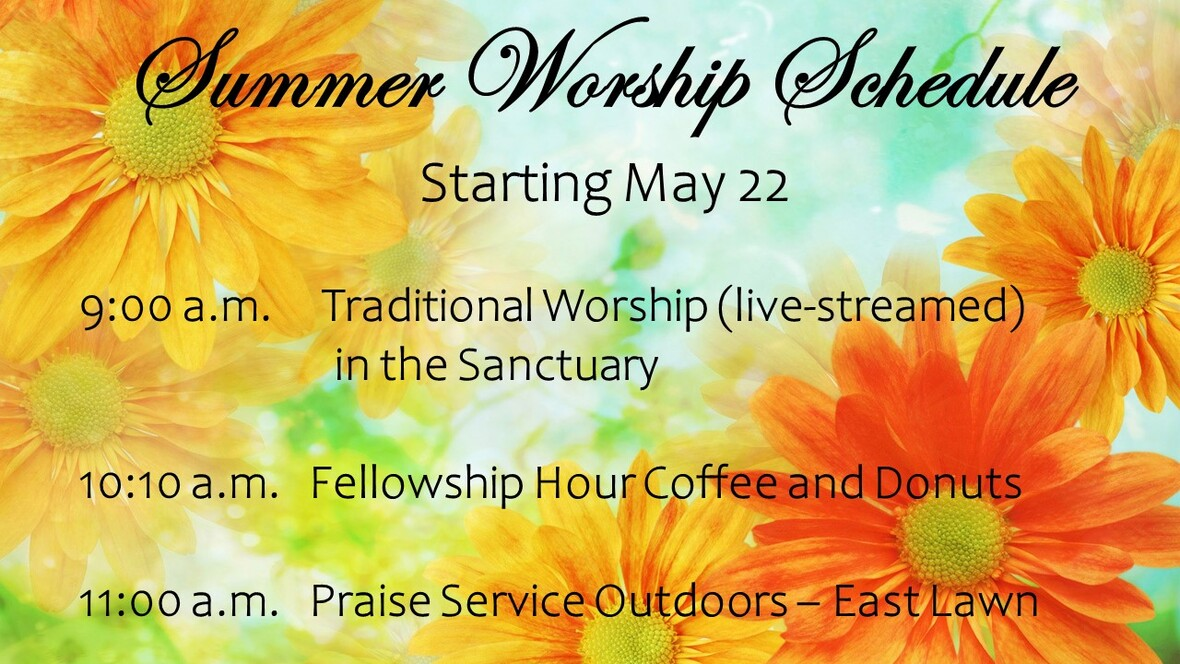table div table+table+table+table+table+table+table+table+table+table+table+table+table+table+table+table+table+table+table+table+table+table+table+table+table+table+table+table+table+table+table+table+table+table+table+table+table div table{width:100%;padding:0}table div table+table+table+table+table+table+table+table+table+table+table+table+table+table+table+table+table+table+table+table+table+table+table+table+table+table+table+table+table+table+table+table+table+table+table+table+table div table img{width:96.23%;padding:0;float:none}table div table+table+table+table+table+table+table+table+table+table+table+table+table+table+table+table+table+table+table+table+table+table+table+table+table+table+table+table+table+table+table+table+table+table+table+table+table div table td{width:100%;padding:0 1.88% 18px}/* styles */table div table+table+table+table+table+table+table+table+table+table+table+table+table+table+table+table+table+table+table+table+table+table+table+table+table+table+table+table+table+table+table+table+table+table+table+table+table+table div table{width:100%;padding:0}table div table+table+table+table+table+table+table+table+table+table+table+table+table+table+table+table+table+table+table+table+table+table+table+table+table+table+table+table+table+table+table+table+table+table+table+table+table+table div table img{width:96.23%;padding:0;float:none}table div table+table+table+table+table+table+table+table+table+table+table+table+table+table+table+table+table+table+table+table+table+table+table+table+table+table+table+table+table+table+table+table+table+table+table+table+table+table div table td{width:100%;padding:0 1.88% 18px}/* styles */## Our St. Mark members:

Carol Kilts, Marsha Carlson, Dennis Pitcock, Linda Vivian, Tim Panjkovich, Rita Olson, Fritzi Panjkovich, Bev Johnson, Beth Johnson, Jack Sowl, Lorine Kortus, Jan Cotter, Diane Swanson, Sherri Lindquist, Bette Patterson, Keith Haggestad.

## Family and friends of St. Mark members:

Becky Belk, Janet Webster, Brock Lawrence, Carrie Nelson, Bill Hart, Roger Clark, Ellie Krepfle, Carl Steingraeber, Don Minnis, Tom Montgomery, Jon Baylander, Debbie Park, John Mortensen, Carolyn Neagle, Sue Flott, Bob Pollack, Jenny Hobby, Bill King, Clyde Webster, Conrad Semmelroth, Nici King, Debbie Adams, Linda Johnson, Eva Love Sherbondy, Norma Comstock, Carolyn King.

## Those serving in the military and their families:

Logan Gehrke, Hunter Haggestad, Tristan Davenport, Scot Hornick,
Bailey Panjkovich, Jim Tammen, Brandon Ballenger, Taylor Eversole.

Strengthen and encourage those in public health services and in the medical profession: care-givers, nurses, attendants, doctors, all who commit themselves to care for the sick and their families.

 table div table+table+table+table+table+table+table+table+table+table+table+table+table+table+table+table+table+table+table+table+table+table+table+table+table+table+table+table+table+table+table+table+table+table+table+table+table+table+table+table+table div table{width:100%;padding:0}table div table+table+table+table+table+table+table+table+table+table+table+table+table+table+table+table+table+table+table+table+table+table+table+table+table+table+table+table+table+table+table+table+table+table+table+table+table+table+table+table+table div table img{width:96.23%;padding:0;float:none}table div table+table+table+table+table+table+table+table+table+table+table+table+table+table+table+table+table+table+table+table+table+table+table+table+table+table+table+table+table+table+table+table+table+table+table+table+table+table+table+table+table div table td{width:100%;padding:0 1.88% 18px}/* styles *//* styles */ Summer Office Hours Monday through Thursday 9:00 A.M. - 3:00 P.M. Friday - 9:00 A.M. - 1:00 P.M. Telephone: 815-398-3557 Publications St. Mark Memos, the weekly e-newsletter, is distributed each Thursday. The deadline for submission is Monday by 3:00 P.M. Please click to send submissions. Worship Services Miss a Sunday at St. Mark? Please visit our website and watch the service online. Please click to watch.
 table div table+table+table+table+table+table+table+table+table+table+table+table+table+table+table+table+table+table+table+table+table+table+table+table+table+table+table+table+table+table+table+table+table+table+table+table+table+table+table+table+table+table+table div table{width:100%;padding:0}table div table+table+table+table+table+table+table+table+table+table+table+table+table+table+table+table+table+table+table+table+table+table+table+table+table+table+table+table+table+table+table+table+table+table+table+table+table+table+table+table+table+table+table div table img{width:96.23%;padding:0;float:none}table div table+table+table+table+table+table+table+table+table+table+table+table+table+table+table+table+table+table+table+table+table+table+table+table+table+table+table+table+table+table+table+table+table+table+table+table+table+table+table+table+table+table+table div table td{width:100%;padding:0 1.88% 18px}/* styles */